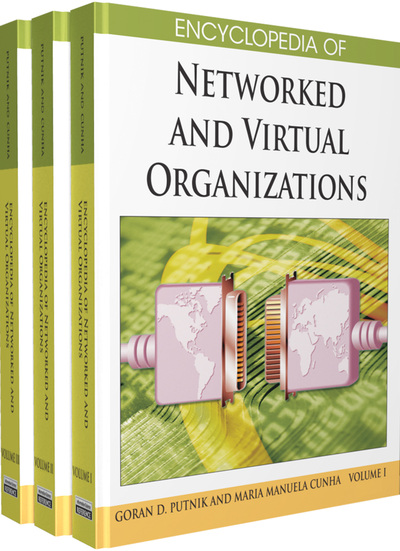# Logistic Models for Symbiosis, Predator-Prey, and Competition

Ricardo Lopez-Ruiz (Universidad de Zaragoza, Spain) and Danièle Fournier-Prunaret (Institut National des Sciences Appliquées, France)
DOI: 10.4018/978-1-59904-885-7.ch111

## Abstract

If one isolated species (corporation) is supposed to evolve following the logistic mapping, then we are tempted to think that the dynamics of two species (corporations) can be expressed by a coupled system of two discrete logistic equations. As three basic relationships between two species are present in nature, namely symbiosis, predator-prey, and competition, three different models are obtained. Each model is a cubic two-dimensional discrete logistic-type equation with its own dynamical properties: stationary regime, periodicity, quasi-periodicity, and chaos. We also propose that these models could be useful for thinking in the different interactions happening in the economic world, as for instance for the competition and the collaboration between corporations. Furthermore, these models could be considered as the basic ingredients to construct more complex interactions in the ecological and economic networks.

## Key Terms in this Chapter

Contact Bifurcation: Bifurcation involving the contact between the boundaries of different regions. For instance, the contact between the boundary of a chaotic attractor and the boundary of its basin of attraction or the contact between a basin boundary and a critical curve LC are examples of this kind of bifurcation.

Chaotic Attractor: A chaotic area, which is attracting.

Basin: The basin of attraction of an attracting set is the set of all points, which converge towards the attracting set.

Neimark-Sacker Bifurcation: Also known as secondary Hopf bifurcation. The physical mark of this bifurcation is the new additional frequency that appears in the time signal.

Immediate Basin: The largest connected part of a basin containing the attracting set.

Flip Bifurcation: Also known as period-doubling bifurcation. The physical trace of this bifurcation is the period doubling (or frequency halving) of the periodic time signal generated by the system.

Attracting: An invariant subset of the plane is attracting if it has a non-zero measure neighbourhood every point of which tends asymptotically to that subset or arrives there in a finite number of iterations.

Chaotic Area: An invariant subset that exhibits chaotic dynamics. A typical trajectory fills this area densely.

## Complete Chapter List

Search this Book:
Reset